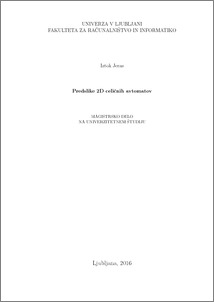# Preimages of 2D cellular automata

Iztok Jeras (2016) Preimages of 2D cellular automata. MSc thesis.Preview
PDF

## Abstract

While computing preimages of 1D cellular automata is a well researched and documented problem, for 2D cellular automata there is less research available. For the 1D problem we know algorithms for counting and listing preimages where computational complexity is a linear function of the size of the problem. It is possible to determine whether a 1D cellular automaton is reversible, and what is the Garden of Eden sequence regular language. For the 2D problem we know a few algorithms, but they are poorly theoretically researched. We also know that the reversibility problem is in general undecidable for 2D cellular automata. The preimage network, first developed for 1D cellular automata, was proved to be a useful tool for explaining algorithms and for constructing proofs. Here I explain how to construct the preimage network for 2D cellular automata. While for the 1D problem this network is a normal graph, for 2D it was extended into the third dimension. Preimages are transformed from paths in the graph in 1D into surfaces on the network in 2D. Edge conditions are transformed from weights for vertices ending a path in the 1D problem into weights for the closed path around a preimage surface in the 2D problem. While developing the algorithm, it proved impossible to count preimages of 2D cellular automata with processing requirements growing linearly with problem size. Instead, processing requirements grow exponentially with the size in one of the dimensions. The described algorithm does not differ much from the existing ones. The cellular automaton is split into rows, the preimage list is first determined for each row from the first to the last. The row results are then combined into the result for the whole 2D problem. In a similar fashion to the 1D approach, the algorithm splits into two passes. In the first pass preimages are counted, in the second optional pass preimages are listed. The second pass is performed in the opposite direction, while rows are also observed from the opposite side. I see the main advantage of the described algorithm in using existing solutions for row processing. Solutions proved to be effective in solving the 1D problem. Using progressive encoding of intermediate solutions also enables reducing memory consumption.

Item Type: Thesis (MSc thesis)
Keywords: cellular automata, preimages, predecessors, ataviser, computational complexity, reversi- bility, Garden of Eden, Conway’s Game of Life, trid, quad
Number of Pages: 37
Language of Content: Slovenian
Mentor / Comentors:
Name and SurnameIDFunction
prof. dr. Branko Šter283Mentor